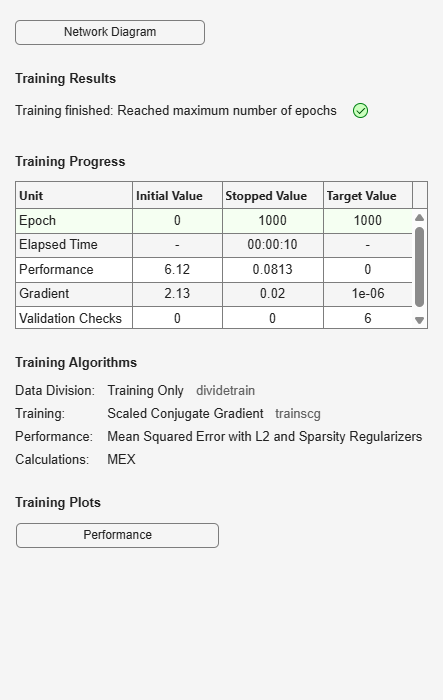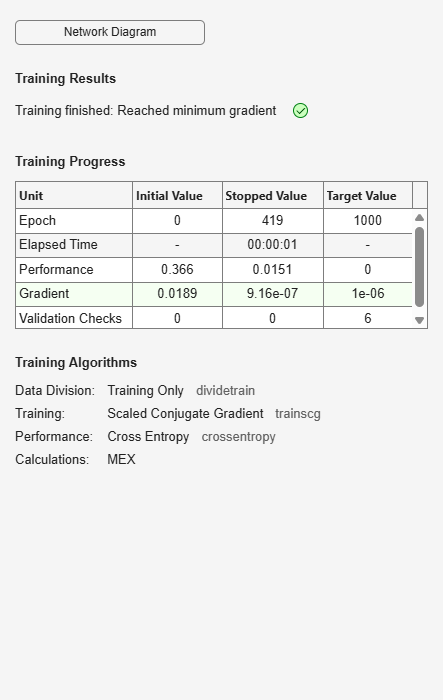# stack

Class: Autoencoder

Stack encoders from several autoencoders together

## Syntax

``stackednet = stack(autoenc1,autoenc2,...)``
``stackednet = stack(autoenc1,autoenc2,...,net1)``

## Description

````stackednet = stack(autoenc1,autoenc2,...)` returns a `network` object created by stacking the encoders of the autoencoders, `autoenc1`, `autoenc2`, and so on.```

example

````stackednet = stack(autoenc1,autoenc2,...,net1)` returns a network object created by stacking the encoders of the autoencoders and the network object `net1`.The autoencoders and the network object can be stacked only if their dimensions match.```

## Input Arguments

expand all

Trained autoencoder, specified as an `Autoencoder` object.

Trained autoencoder, specified as an `Autoencoder` object.

Trained neural network, specified as a `network` object. `net1` can be a softmax layer, trained using the `trainSoftmaxLayer` function.

## Output Arguments

expand all

Stacked neural network (deep network), returned as a `network` object

## Examples

expand all

`[X,T] = iris_dataset;`

Train an autoencoder with a hidden layer of size 5 and a linear transfer function for the decoder. Set the L2 weight regularizer to 0.001, sparsity regularizer to 4 and sparsity proportion to 0.05.

```hiddenSize = 5; autoenc = trainAutoencoder(X, hiddenSize, ... 'L2WeightRegularization', 0.001, ... 'SparsityRegularization', 4, ... 'SparsityProportion', 0.05, ... 'DecoderTransferFunction','purelin');```Extract the features in the hidden layer.

`features = encode(autoenc,X);`

Train a softmax layer for classification using the `features` .

`softnet = trainSoftmaxLayer(features,T);`Stack the encoder and the softmax layer to form a deep network.

`stackednet = stack(autoenc,softnet);`

View the stacked network.

`view(stackednet);`## Tips

• The size of the hidden representation of one autoencoder must match the input size of the next autoencoder or network in the stack.

The first input argument of the stacked network is the input argument of the first autoencoder. The output argument from the encoder of the first autoencoder is the input of the second autoencoder in the stacked network. The output argument from the encoder of the second autoencoder is the input argument to the third autoencoder in the stacked network, and so on.

• The stacked network object `stacknet` inherits its training parameters from the final input argument `net1`.

## Version History

Introduced in R2015b